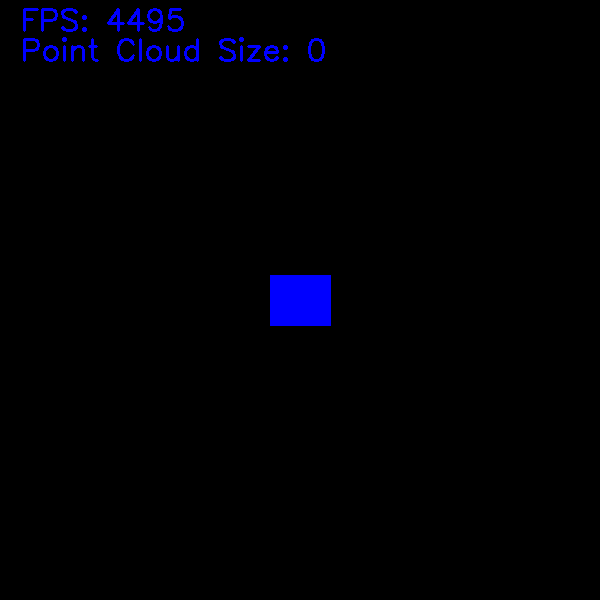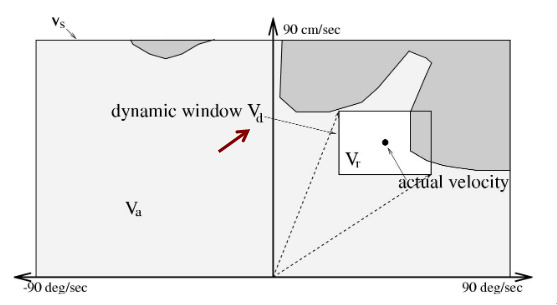dynamic-window-approach
pypi i dynamic-window-approach

# dynamic-window-approach

## The Dynamic Window Approach (DWA) planning algorithm written in C with Python Bindings

### by Göktuğ Karakaşlı

pypi i dynamic-window-approach

# Dynamic Window Approach

2D Dynamic Window Approach  Motion Planning algorithm written in C with Python Bindings.## Online Demo

https://goktug97.github.io/dwa/

## Requirements

### Python

• Python >= 3.6
• cython
• numpy
• cv2 (Optional for the demo)

• SDL
• OpenGL

## Installation

You can directly install Python bindings without compiling the library.

### Compile and Install C Library

``````git clone https://github.com/goktug97/DynamicWindowApproach
cd DynamicWindowApproach
mkdir build
cd build
cmake ..
make
make install
# Optional: Build Demo
make demo
``````

### Install Python Bindings

#### PyPI

``````pip3 install dynamic-window-approach --user
``````

#### Source

``````git clone https://github.com/goktug97/DynamicWindowApproach
cd DynamicWindowApproach
python3 setup.py install --user
``````

## Usage

• Only function you need to run to plan the next move is the planning function. Rest of the code for both C and Python examples are just to create simulation environment and GUI. The 2 examples that you can find in examples folder is the same demo but implemented using different libraries for visualization.

• The Python example uses OpenCV and you can run it by executing `python3 demo.py` in the examples folder.

• The C example uses OpenGL and SDL and you can run it by executing `./demo` in bin folder. The bin folder is created after the compile so if you didn't compile the demo while installing the library. Go to `build` directory and run `make demo`.

## Documentation

While the planning function is the only function that a user needs to call for the planning, all of the functions are exposed to the user for both C and Python for no reasons.

### Structs and Classes

If you are using Python bindings, you don't need to use any of these classes except Config. The functions accept built-in or numpy types. The functions create required classes inside for easy usage. For example a snippet from the planning function;

``````cdef float x, y, yaw, v , w, gx, gy
cdef PointCloud _point_cloud = PointCloud(point_cloud)
x, y, yaw = pose
v, w = velocity
gx, gy = goal
cdef Pose _pose = Pose(Point(x, y), yaw)
cdef Velocity _velocity = Velocity(v, w)
cdef Point _goal = Point(gx, gy)
``````
• Structs are for C
• Classes are for Python

#### Rect

• struct Rect

• Given center of the robot is (0, 0)
• Parameters:
• xmin - floating-point minimum x-coordinate of the robot.
• ymin - floating-point minimum y-coordinate of the robot.
• xmax - floating-point maximum x-coordinate of the robot.
• ymax - floating-point maximum y-coordinate of the robot.

#### Config

• struct Config

• Parameters:
• maxSpeed - floating-point maximum linear speed robot can reach [m/s]
• minSpeed - floating-point minimum linea speed robot can fall [m/s]
• maxYawrate - floating-point maximum angular spped robot can reach [yaw/s]
• maxAccel - floating-point maximum linear acceleration robot can reach [m/ss]
• maxdYawrate - floating-point maximum angular acceleration robot can reach [yaw/ss]
• velocityResolution - floating-point linear speed resolution [m/s]
• yawrateResolution - floating-point angular speed resolution [m/s]
• dt - floating-point time change [s]
• predictTime - floating-point simulation time [s]
• clearance - floating-point clearance cost weight
• velocity - floating-point velocity cost weight
• base - Rect
• class Config

``````Config(float max_speed, float min_speed,
float max_yawrate, float max_accel, float max_dyawrate,
float velocity_resolution, float yawrate_resolution, float dt,
float predict_time, float heading, float clearance, float velocity,
list base)
``````

#### Velocity

• struct Velocity

• Parameters:
• linearVelocity - floating-point linear velocity of the robot [m/s]
• angularVelocity - floating-point angular velocity of the robot [yaw/s]
• class Velocity

``````Velocity(float linear_velocity, float angular_velocity)
``````

#### Point

• struct Point

• Parameters:
• x – floating-point x-coordinate of the point.
• y – floating-point y-coordinate of the point.
• class Point

``````Point(float x, float y)
``````

#### PointCloud

• struct PointCloud

• int size
• Number of points.
• Point *points
• class PointCloud

``````PointCloud(np.ndarray[np.float32_t, ndim=2] point_cloud)
``````

#### Pose

• struct Pose

• Point point
• Coordinate of the robot.
• float yaw
• Angle of the robot.
• class Pose

``````Pose(Point point, float yaw)
``````

#### DynamicWindow

• struct DynamicWindow

• int nPossibleV:
• Number of linear velocities in the Dynamic Window.
• float *possibleV:
• int nPossibleW:
• Number of angular velocities in the Dynamic Window
• float *possibleW:
• class DynamicWindow

``````DynamicWindow(tuple velocity, Config config)
``````

### Functions

#### planning

Calculates best linear and angular velocities given the state. Only required function to use this library.

• C

``````Velocity planning (Pose pose, Velocity velocity, Point goal, PointCloud *pointCloud, Config config);
``````
• Python

``````linear_velocity, angular_velocity = planning(pose, velocity, goal, point_cloud, config)
``````
• Parameters:
• pose: tuple: (x, y, yaw)
• velocity: tuple: (Linear Velocity, Angular Velocity)
• goal: tuple: (x, y)
• point_cloud: Numpy Array of shape (N, 2) and type np.float32
• config: Config

#### createDynamicWindow

Given robot configuration and current velocities, calculates DynamicWindow. The memory is allocated dynamically inside of the function and must be freed using freeDynamicWindow function.

• C

``````void createDynamicWindow(Velocity velocity, Config config, DynamicWindow **dynamicWindow);
``````
• Python

DynamicWindow class is used to create a DynamicWindow instance.

``````dw = dwa.DynamicWindow(velocity, config):
print(dw.possible_v, dw.possible_w)
``````
• Parameters:
• velocity: tuple: (Linear Velocity, Angular Velocity)
• config: Config class#### freeDynamicWindow

Free dynamically allocated memory.

• C

``````void freeDynamicWindow(DynamicWindow *dynamicWindow);
``````
• Python

Handled by the DynamicWindow class. See below.

``````def __dealloc__(self):
if self.thisptr is not NULL:
cdwa.freeDynamicWindow(self.thisptr)

``````

#### motion

Given current position and velocities, calculates next position after given dt using differential drive motion model. Can be used to simulate motion in a simulated environment.

• C

``````Pose motion(Pose pose, Velocity velocity, float dt);
``````
• Parameters:
• Python

``````x, y, yaw = motion(pose, velocity, dt)
``````
• Parameters:
• pose: tuple: (x, y, yaw)
• velocity: tuple: (Linear Velocity, Angular Velocity)

#### calculateVelocityCost

• C

``````float calculateVelocityCost(Velocity velocity, Config config);
``````
• Python

``````velocity_cost = calculate_velocity_cost(velocity, config)
``````
• Parameters:
• velocity: tuple: (Linear Velocity, Angular Velocity)
• config: Config

• C

``````float calculateHeadingCost(Pose pose, Point goal);
``````
• Python

``````heading_cost = calculate_heading_cost(pose, goal)
``````
• Parameters:
• pose: tuple: (x, y, yaw)
• goal: tuple: (x, y)

#### calculateClearanceCost

• C

``````float calculateClearanceCost(Pose pose, Velocity velocity, PointCloud *pointCloud, Config config);
``````
• Python

``````clearance_cost = calculate_clearance_cost(pose, velocity, point_cloud, config)
``````
• Parameters:
• pose: tuple: (x, y, yaw)
• velocity: tuple: (Linear Velocity, Angular Velocity)
• point_cloud: Numpy Array of shape (N, 2) and type np.float32
• config: Config

#### createPointCloud

Given a size, creates a PointCloud. Must be freed using freePointCloud.

• C

``````PointCloud* createPointCloud(int size);
``````
``````for (int i = 0; i < pointCloud->size; ++i) {
pointCloud->points[i].x = 0.0
pointCloud->points[i].y = 0.0
}
``````
• Parameters:
• size: int
• Python

PointCloud class is used to create a PointCloud instance. All functions in python accepts numpy array instead of PointCloud instance. The PointCloud instance is created inside of the function.

``````size = 600
point_cloud = np.zeros((size, 2), dtype=np.float32)
point_cloud = dwa.PointCloud(point_cloud)
``````

#### freePointCloud

• C

``````void freePointCloud(PointCloud* pointCloud);
``````
• Python

Handled by the PointCloud class. See below.

``````def __dealloc__(self):
if self.thisptr is not NULL:
cdwa.freePointCloud(self.thisptr)
``````

30

1yr ago

1

3

0

### OPEN PRs

0
VersionTagPublished
1.1.1
2yrs ago
1.1.0
3yrs ago
1.0.3
3yrs ago
1.0.2
3yrs ago